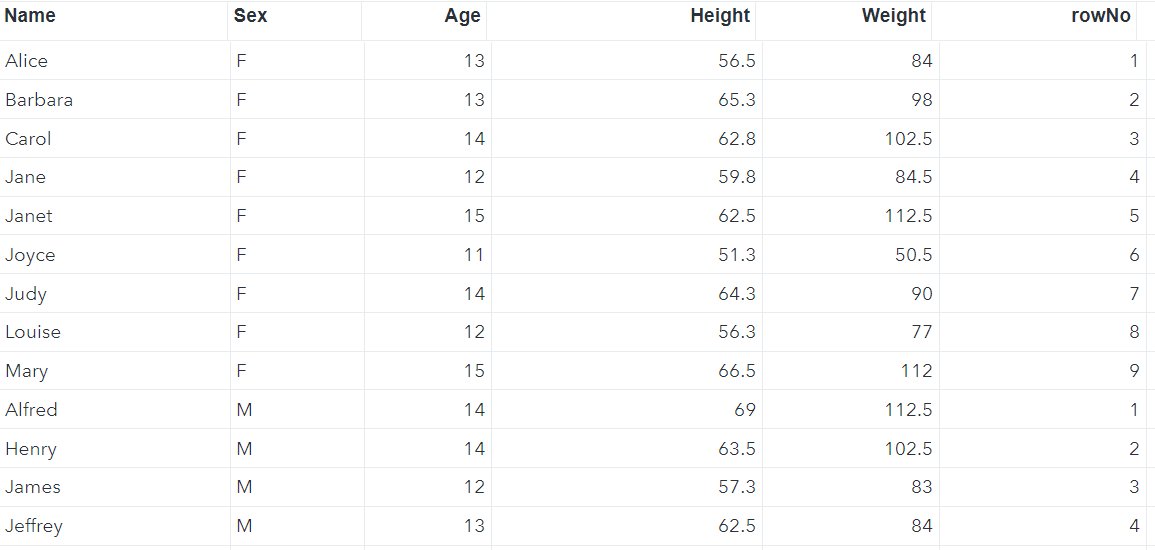# 4 Ways to Add Row Numbers in SAS

This tutorial explains various ways to add rows numbers in SAS, along with examples.

Method 1: _N_ Variable

In SAS, we can use the automatic variable `_N_` to generate row numbers.

```data want;
rowNo = _N_;
set sashelp.class;
run;
```

We have created a new variable called "rowNo" that stores row numbers in the new dataset named "want".Method 2: monotonic() function in Proc SQL

We can use the `monotonic()` function in PROC SQL to generate row numbers in SAS.

```proc sql noprint;
create table want as
select  monotonic() as rowNo, *
from sashelp.class;
quit;
```
Method 3: NUMBER option in Proc SQL

In Proc SQL, there is an option called NUMBER that creates a column called ROW, which is the row (or observation) number of the data.

```ods listing;
ods output SQL_Results=want;
proc sql number;
select * from sashelp.class;
quit;
```
The limitation of this method is that it does not work when you don't want to print the dataset.
Method 4: Add Row Numbers by Group

Suppose you want to generate row numbers by one or more groups. In this case, we can use the FIRST.variable to initialize the row numbers to 1 for the first observation within each category of a group. For the other observations within the same category, the row number is incremented by 1.

First we need to sort the dataset by the categorical variable using PROC SORT.

```/* Step 1: Sort Dataset by Sex (categorical variable)*/
proc sort data=sashelp.class out=class;
by sex;
run;

/* Step 2: Add row number by Sex*/
data want;
set class;
by sex;
if first.sex then rowNo=1;
else rowNo+1;
run;
```

The output dataset has a new variable called "rowNo" that contains the row number within each category.Related Posts